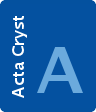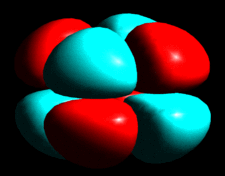# issue contentsFOUNDATIONSADVANCES
ISSN: 2053-2733

# July 2001 issueCover illustration: Graphical representation of density modification due to third-order terms b113 = -b223 (point group) in the Gram-Charlier series expansion of a Gaussian atomic probability density function. Courtesy of W. F. Kuhs.

## research papers

### Geometrical theory of triple junctions of CSL boundaries

The coincidence site lattice theory of grain boundaries is extended to triple junctions. It provides a basis for analyzing triple-junction structure.

### Introduction to a general crystallography

Extended crystallographic groups, which may include hyperbolic rotations as well, are defined in terms of a faithful integral representation of a permutation group of atomic positions. This formulation unifies in a general frame the crystallographic characterization of periodic and aperiodic crystals and even of some single molecules.

### Approximate solution of the Takagi–Taupin equations for a semi-infinite crystal in the three-beam Laue–Laue case

By using the Takagi–Taupin theory, an analytical expression for the perturbation of the integrated primary diffracted power, restricted to small crystal thicknesses, is obtained. The result is found to be in perfect agreement with the full numerical solution of the fundamental equations.

### Critical examination of the radial functions in the Hansen–Coppens multipole model through topological analysis of primary and refined theoretical densities

An aspherical atom (multipole) refinement model with two sets of atomic deformation functions on each atom is tested with ab initio crystal structure factors on four different molecular crystals. The flexible model provides a quantitatively improved fit to the density and its topological parameters in the bonding regions between atoms.

### A proposal for a transition mechanism from the diamond to the lonsdaleite type

A new model for the phase transformation between the diamond and the lonsdaleite type is proposed. It makes use of group–subgroup relations and describes the phase transformation as a deformation of a homogeneous sphere packing with three contacts per sphere in space group Pnna.

### The method of joint probability distribution functions applied to MAD techniques. The centric case

The joint probability distribution functions method is applied to estimate phases for MAD data.

### Phase determination and extension using X-ray multiple diffraction and the maximum-entropy method

The extension of the phases of the structure factors of the organic crystal C25H25NO2 from 77 starting individual phases derived from multiple diffraction is realized using the maximum-entropy method. More than 100 new phases are generated and an electron-density map at 1.97 Å is deduced.

### Rapid neutron-diffraction data collection for hydrogen-bonding studies: application of the Laue diffractometer (LADI) to the case study zinc (tris­)thiourea sulfate

The successful application of the newly developed image-plate neutron Laue diffractometer, LADI, for rapid hydrogen-bonding characterization is reported. The case study concerns the non-linear optical material zinc (tris)thiourea sulfate and results from 12 h data collection compare very well with those obtained over 135 h using a crystal 13 times larger in volume and a monochromatic four-circle diffractometer at the same neutron source.

### Latent symmetry and its group theoretical determination

The concept of latent symmetry of a component of a composite object is defined and a method is given for its determination.

### Derivation of a scoring function for crystal structure prediction

A simple learning machine is applied to the Cambridge Structural Database to derive a scoring function. The function is compared to a common force field and is successfully validated for crystal structure prediction on several hundreds of crystal structures.

### Fast differentiation algorithm and efficient calculation of the exact matrix of second derivatives

Algorithms to calculate rapidly the exact matrix of the second derivatives of crystallographic functionals are described.

### The joint probability distributions of structure-factor doublets in displacive incommensurately modulated structures and their applicability to direct methods

The joint probability distribution of the phase sum of two first-order satellite reflections in structures with an incommensurately displacive modulation is derived under the assumption that the phase of the associated main reflection can be calculated from the known main (or averaged) structure. The functional (dis-)similarities with conventional direct methods, employing normalized structure factors and the Cochran distribution, are assessed and test results are presented showing a significant improvement of the direct-methods phase-sum statistics when the new distribution is used.

## short communications

### Inversion of dynamical electron scattering to obtain the crystal potential using data from two thicknesses

A method is given to invert the multiple scattering in many-beam dynamical electron scattering to obtain the crystal potential.

### The Fedorov algorithm revised

All the simple 12- to 20-hedra with no triangular and quadrilateral facets (118 in common) are calculated in the Schlegel projections.

## book reviews

Acta Cryst. (2001). A57, 478
doi: 10.1107/S0108767301005980

## books received

Acta Cryst. (2001). A57, 478
doi: 10.1107/S0108767301006882

### Worked examples in the geometry of crystals.

Follow Acta Cryst. A